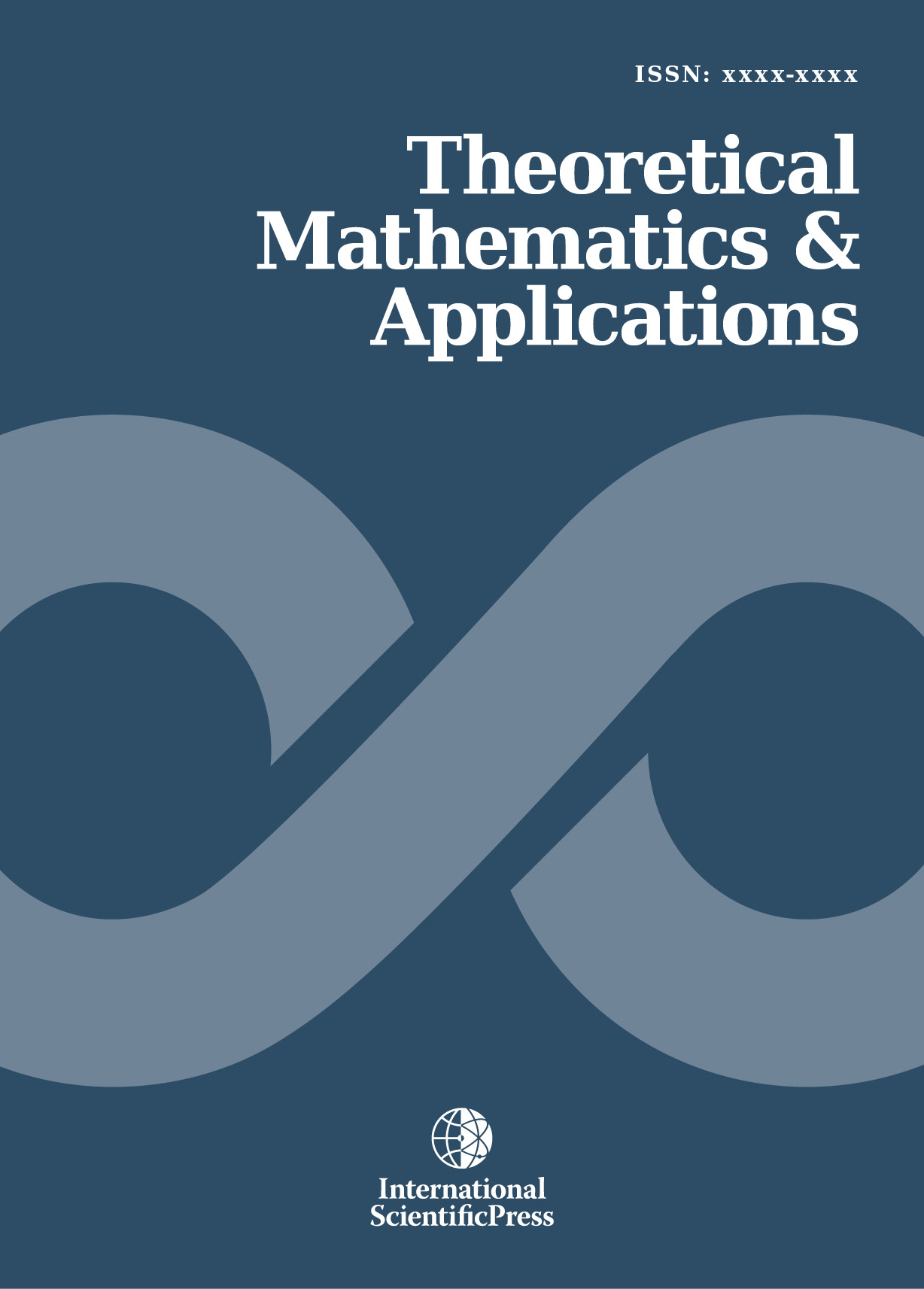# Theoretical Mathematics & Applications

#### A New Class of Generalized Power Lindley Distribution with Applications to Lifetime Data

•[ Download ]
• Times downloaded: 1986
• Abstract

A new class of distribution called the beta-exponentiated power Lindley (BEPL) distribution is proposed. This class of distributions includes the Lindley (L), exponentiated Lindley (EL), power Lindley (PL), exponentiated power Lindley (EPL), beta-exponentiated Lindley (BEL), beta-Lindley (BL), and beta-power Lindley distributions (BPL) as special cases. Expansion of the density of BEPL distribution is obtained. Some mathematical properties of the new distribution including hazard function, reverse hazard function, moments, mean deviations, Lorenz and Bonferroni curves are presented. Entropy measures and the distribution of the order statistics are given. The maximum likelihood estimation technique is used to estimate the model parameters. Finally, real data examples are discussed to illustrate the usefulness and applicability of the proposed distribution.ISSN: 1792-9687 (Print)
1792-9709 (Online)